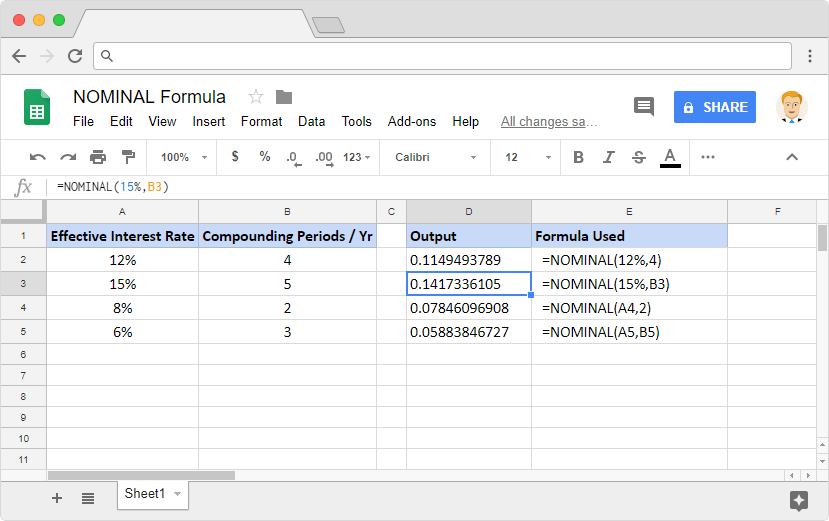# How to use the NOMINAL formula in Google SheetsWith the NOMINAL interest rate formula in Google Sheets, we can calculate the annual nominal interest rate. We just need to have an effective interest rate per annum and the number of compounding periods per year.

The nominal interest rate usually refers to the interest rate before taking inflation into account. But, the nominal interest rate can also refer to the stated or advertised interest rate on a loan. Without taking into consideration any fees or compounding of interest, that is.

### Syntax

NOMINAL(effective_rate, periods_per_year)

• effective_rate – is the effective interest rate per year.
• periods_per_year – are the number of compounding periods per year.

### Usage: NOMINAL interest rate formula in Google Sheets

This is relatively a simple formula to master. Nevertheless, let us try our hands with a few examples as shown in the screenshot below. They help us attain more clarity as to how the formula behaves with a different set of input values.The NOMINAL interest rate formula is used on column D, whereas the columns A and B have the input source values on them. For ease of reference, we have included the formulas used on column E. They correspond to the adjacent output values on column D.

We will observe that the formula accepts numeric values. But from the snapshot above, we see that it has no problem accepting references to the cells that have these numeric values.